# Families of Sets and `Generalized' Set Operations

### Finite Set Operations

The operations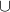and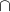apply to two sets; we can however use them to combine more than one set. If we have a finite collection of sets, we write

(1)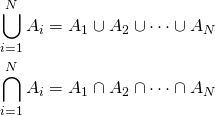Activity. Give a better definition of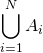and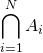. (Hint. What did we say about definitions involving?)

De Morgan's Laws. For any finite collection of sets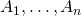,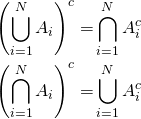Exercise. Prove this version of de Morgan's Laws.

### Transfinite Operations

There is no reason to restrict our attention to {\em finite} collections of sets. For example, we might consider

the collection of intervals of the form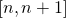, whereis allowed to be an natural number,

or perhaps

the set of all disks centered at the origin

each of which consists of an infinite number of sets. We usually use set-builder notation to write something like

(2)or

(3)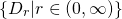to emphasize that what we are dealing with is a(n infinite) set of sets. (Here we have written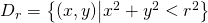for the disk of radius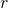centered at the origin.) We call the set to the right of the pipe the index set: in these collections  the index sets are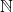and the interval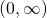, respectively.

We can define unions and intersections of such infinite collections of sets by recalling that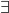acts like a souped-up version of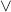and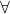acts like a souped-up version of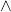.

Given a collection of sets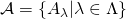, we set

Definition.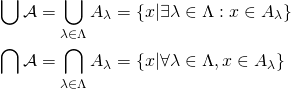E.g.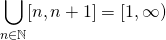Proof. Let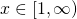. Then there is some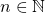with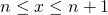, so. Thus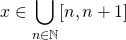.

Now let. Then there is somewith. But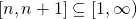, so.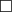E.g.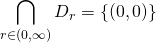Proof. Certainly the origin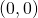is in each disk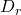. We will show it is the only such point.

Consider any point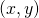in the plane. Let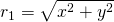be the distance betweenand the origin. Then if we select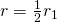, we see that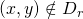. Thuscannot be in every.

de Morgan's Laws. For any collection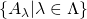, we have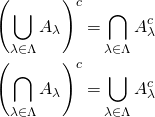Exercise. Prove this version of de Morgan's Laws.

Exercise. What is each of the following sets?

1.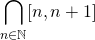2.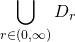3.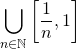4.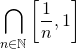Exercise Express each set below as either a generalized union or a generalized intersection, and also express it in set-builder notation.

1.2.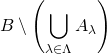3.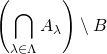4.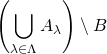Published in sets.